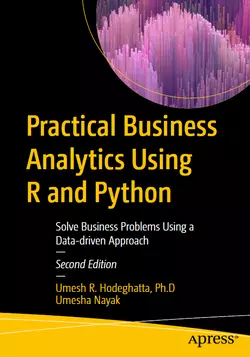# Practical Business Analytics Using R and Python, 2nd Edition### eBook Details:

• Paperback: 731 pages
• Publisher: WOW! eBook; 2nd edition (April 4, 2023)
• Language: English
• ISBN-10: 1484287533
• ISBN-13: 978-1484287538

### Practical Business Analytics Using R and Python: Solve Business Problems Using a Data-driven Approach, 2nd Edition

This book illustrates how data can be useful in solving business problems. It explores various analytics techniques for using data to discover hidden patterns and relationships, predict future outcomes, optimize efficiency and improve the performance of organizations. You’ll learn how to analyze data by applying concepts of statistics, probability theory, and linear algebra. In this new edition, both R and Python are used to demonstrate these analyses. Practical Business Analytics Using R and Python, 2nd Edition also features new chapters covering databases, SQL, Neural networks, Text Analytics, and Natural Language Processing.

Part one begins with an introduction to analytics, the foundations required to perform data analytics, and explains different analytics terms and concepts such as databases and SQL, basic statistics, probability theory, and data exploration. Part two introduces predictive models using statistical machine learning and discusses concepts like regression, classification, and neural networks. Part three covers two of the most popular unsupervised learning techniques, clustering and association mining, as well as text mining and natural language processing (NLP). The book concludes with an overview of big data analytics, R and Python essentials for analytics including libraries such as pandas and NumPy.

• Master the mathematical foundations required for business analytics
• Understand various analytics models and data mining techniques such as regression, supervised machine learning algorithms for modeling, unsupervised modeling techniques, and how to choose the correct algorithm for analysis in any given task
• Use R and Python to develop descriptive models, predictive models, and optimize models
• Interpret and recommend actions based on analytical model outcomes

Upon completing this Practical Business Analytics Using R and Python, 2nd Edition book, you will understand how to improve business outcomes by leveraging R and Python for data analytics.